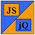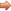# JavaScript & jQuery Tutorials

JS HomeJS AdvancedMath

## Math

The `Math` global object is a static object which means we cannot instantiate objects of type `Math`. All the properties and methods of this class are also static and so we access these using syntax such as `Math.aPropertyName` or `Math.aMethodName`.

In this lesson we explore some of the properties and methods of the `Math` object with examples of their usage.

### `Math` Properties.

Let's look at the properties available for use with the `Math` object.

``````
var mathProps = new Array(8);

/*
* Store properties in an array.
*/
mathProps = Math.E; // Euler's constant
mathProps = Math.LN2; // Natural log of 2
mathProps = Math.LN10; // Natural log of 10
mathProps = Math.LOG2E; // Base 2 log of E
mathProps = Math.LOG10E; // Base 10 log of E
mathProps = Math.PI; // Circumference to diameter ratio of a circle
mathProps = Math.SQRT1_2; // Square root of 1/2
mathProps = Math.SQRT2; // Square root of 2

``````

Press the button below to action the above code:

### Some `Math` Methods.

Let's look at some examples of `Math` methods. For a complete list of `Math` methods and their usages go to the reference section of the site.

``````
var mathMethods = new Array(5);

/*
* Store results in an array.
*/
mathProps = Math.max(10,50,30,12); // Return largest number
mathMethods = Math.min(10,50,30,12); // Return smallest number
mathMethods = Math.cos(25); // Return cosine of a number
mathMethods = Math.sin(25); // Return sine of a number
mathMethods = Math.sqrt(80); // Return positive square root of a number

``````

Press the button below to action the above code:

## Lesson 4 Complete

In this lesson we looked at the `Math` static global object and some of its properties and methods.

## What's Next?

In the next lesson we look at elegant object creation using prototyping.